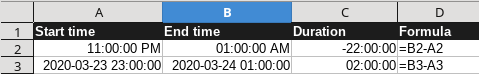# Summing Hours as duration not correct

I am having a time sheet where it monitors the start date and time with the end date and time .
I have successfully managed to subtract the end time from the start time and get the duration .
I want to calculate the duration and I am using the sum for that .
I have formatted my duration cells to be of [H]:MM:SS
and my start and end time formats are HH:MM:SS
however when summing up the duration the number is not correct when the duration is passing midnight :
An example:
from 23:00:00 (11:00 PM ) to 01:00:00 (01:00 AM)
This 2 hours duration is not summed

Please edit your question and attach a sample file, so someone can test the issue.

I want to calculate the duration and I am using the sum

I don’t understand that - a duration isn’t the sum but the difference of two times. And of course you’ll get -22:00 hours for the duration, if you don’t tell LibreOffce that 23:00:00 (11:00 PM ) is meant to be a time of the day before the of day of time 01:00:00 (01:00 AM).When calculating the duration, you took the first step correctly - the difference between the end time and the start time.

If you use the example of colleagues @Opaque formula that he showed

``````=B2-A2
``````

Now just adjust the result for cases where midnight falls into the interval

``````=B2-A2+(IF(CURRENT()<0;1;0))
``````

If two or more midnight fall in the interval, you will not be able to get the correct result without indicating the date

I’d rather use `=B2-A2+(A2>B2)` than CURRENT().